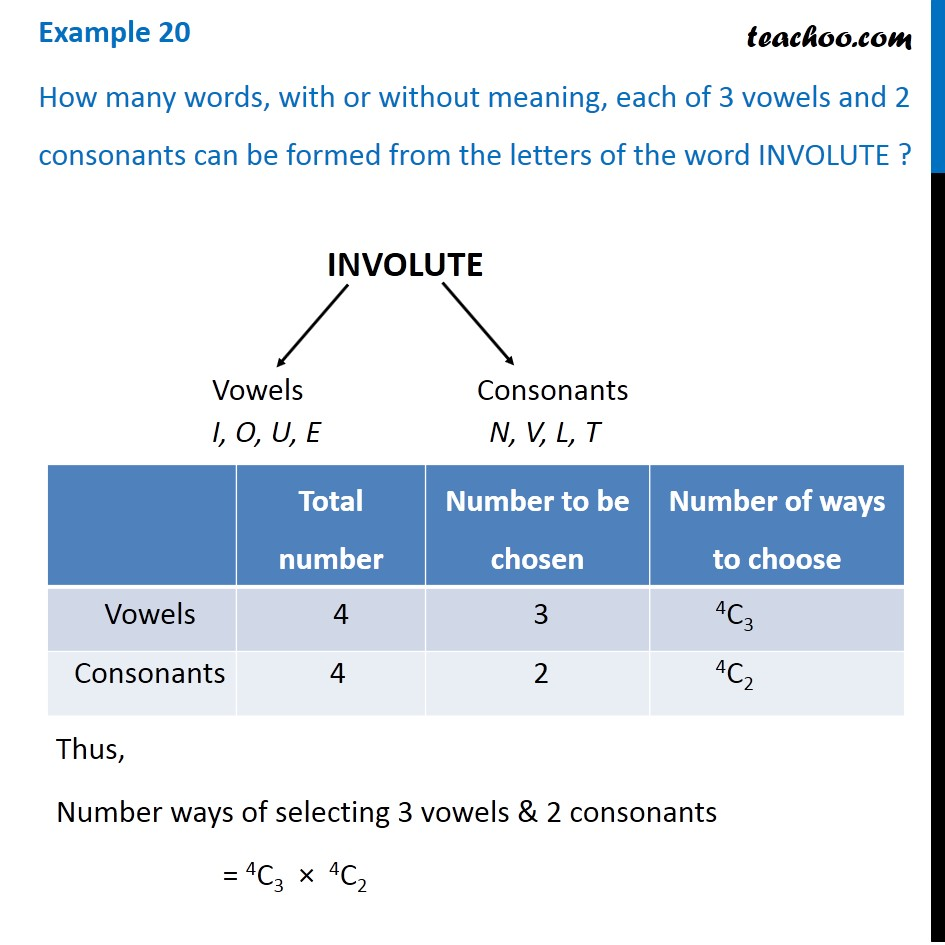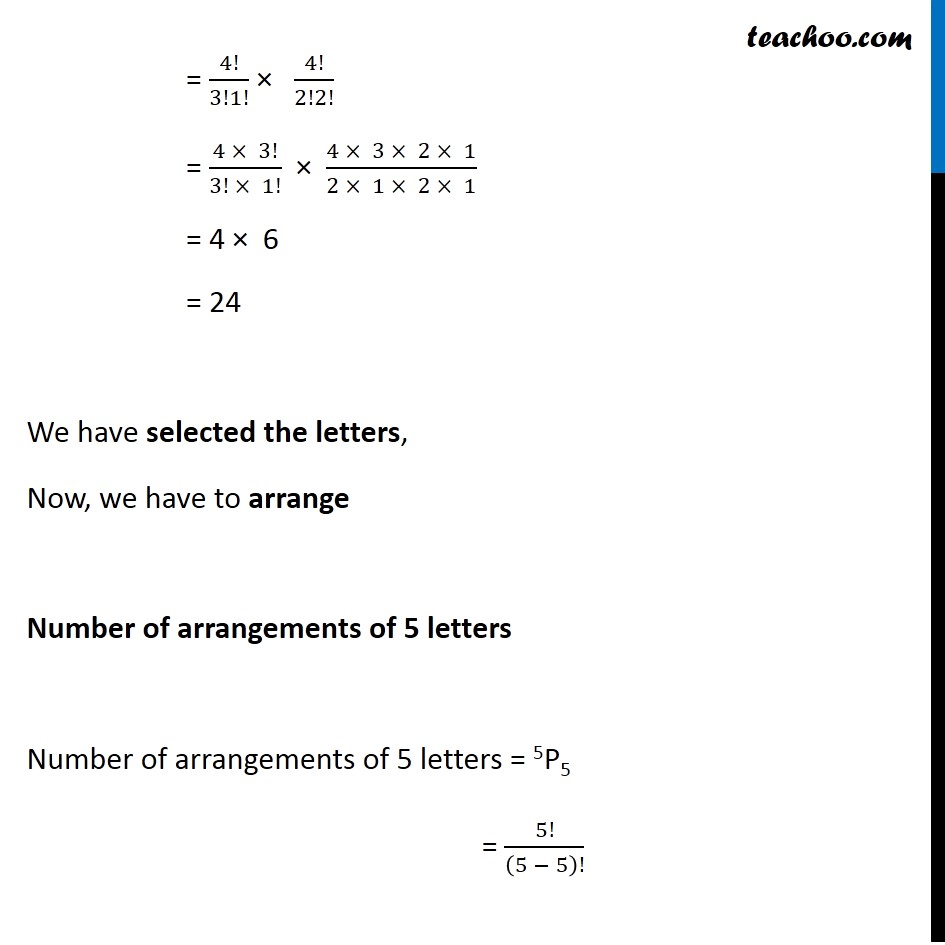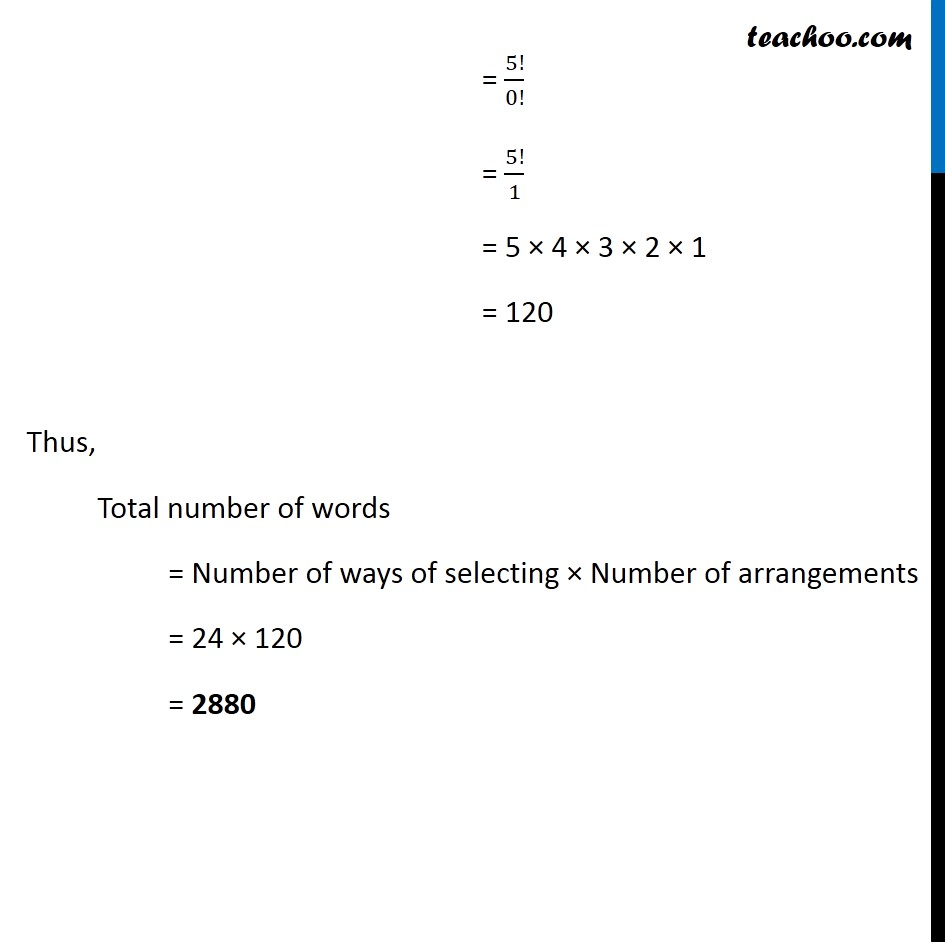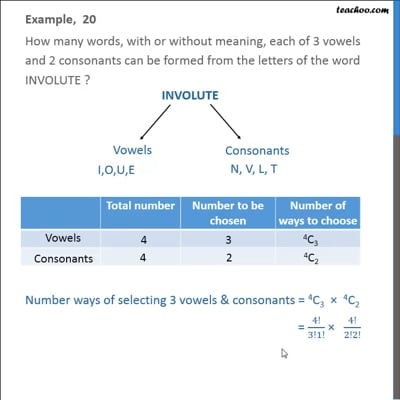Examples

Chapter 7 Class 11 Permutations and Combinations (Term 2)
Serial order wiseThis video is only available for Teachoo black users

### Transcript

Example 20 How many words, with or without meaning, each of 3 vowels and 2 consonants can be formed from the letters of the word INVOLUTE ? Thus, Number ways of selecting 3 vowels & 2 consonants = 4C3 × 4C2 = 4!/3!1! × 4!/2!2! = (4 × 3!)/(3! × 1!) × (4 × 3 × 2 × 1)/(2 × 1 × 2 × 1) = 4 × 6 = 24 We have selected the letters, Now, we have to arrange Number of arrangements of 5 letters Number of arrangements of 5 letters = 5P5 = 5!/(5 − 5)! = 5!/0! = 5!/1 = 5 × 4 × 3 × 2 × 1 = 120 Thus, Total number of words = Number of ways of selecting × Number of arrangements = 24 × 120 = 2880